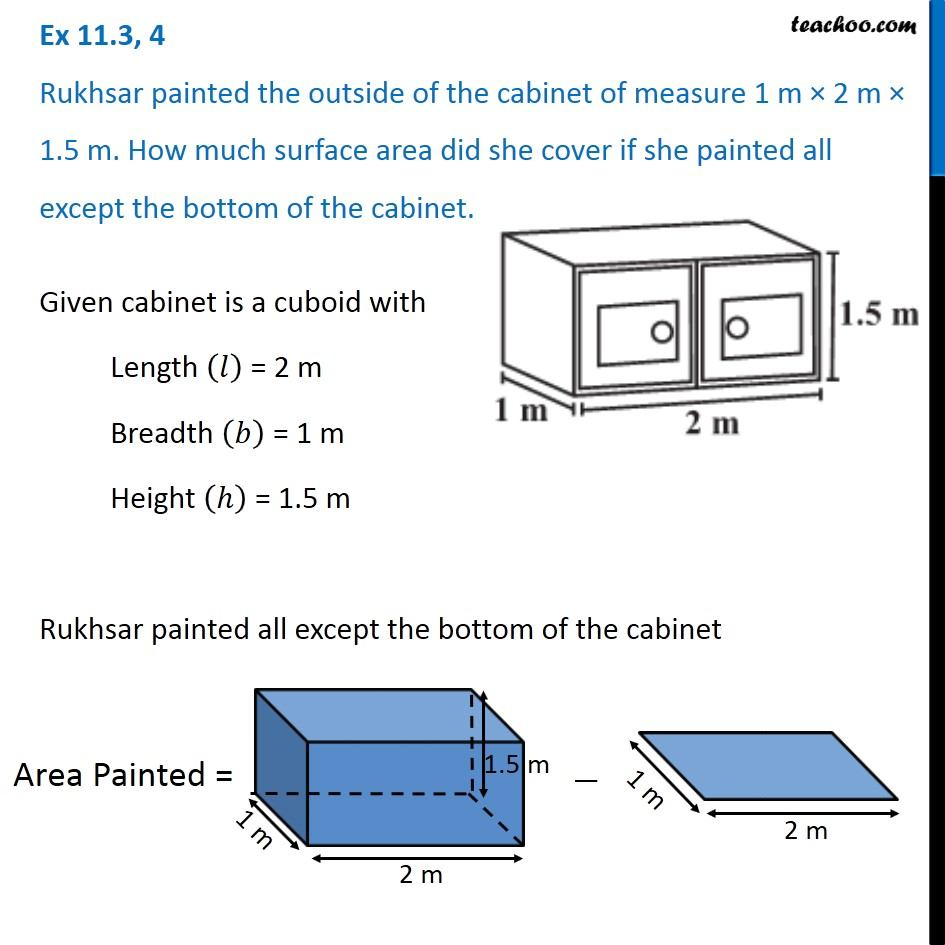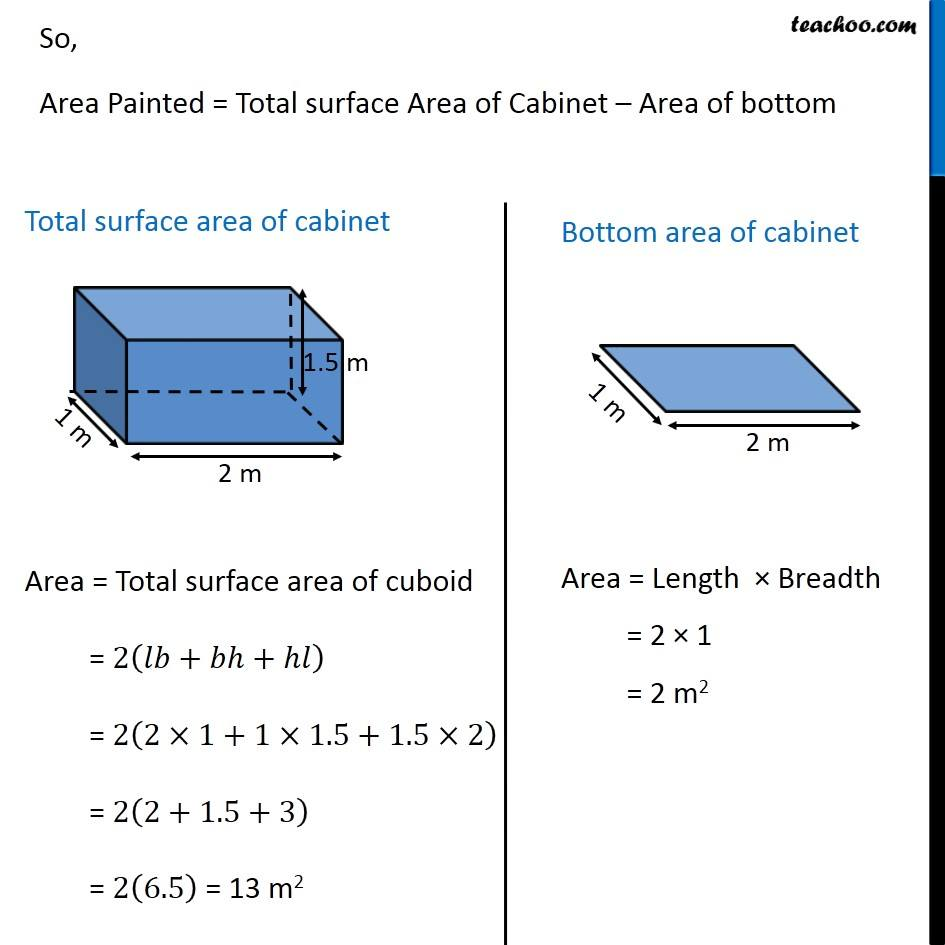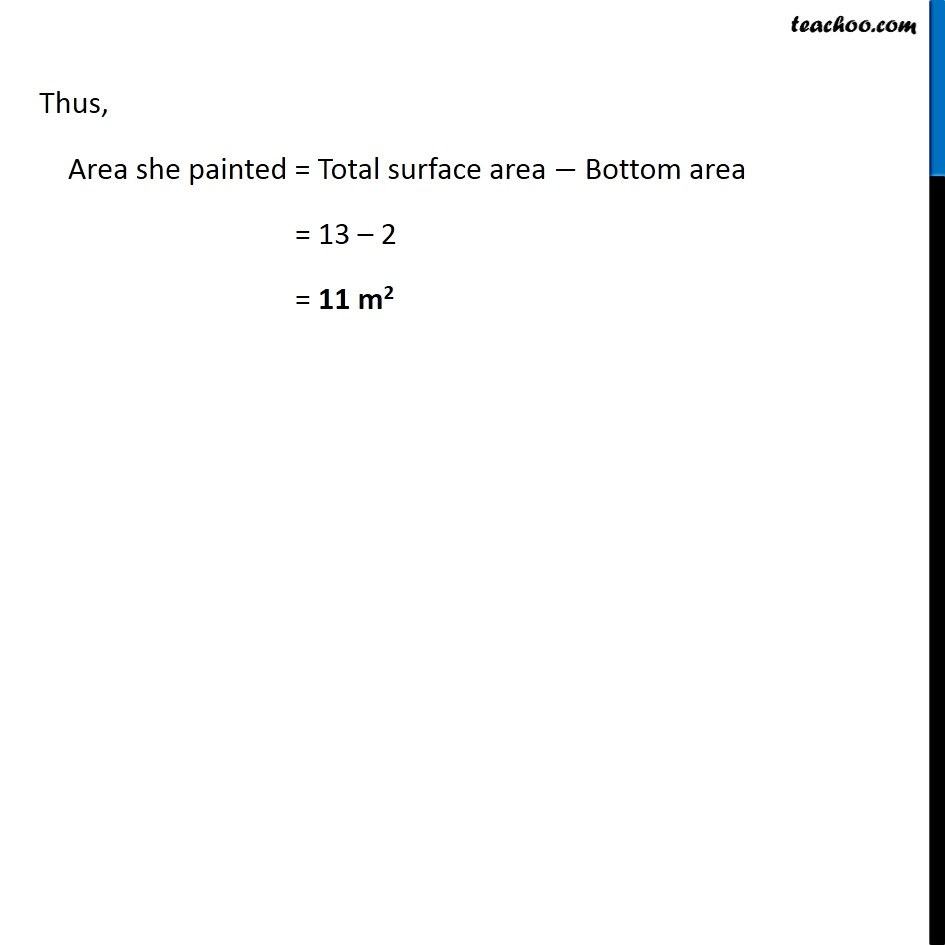Surface Area of Cuboid

Chapter 11 Class 8 Mensuration
Concept wiseIntroducing your new favourite teacher - Teachoo Black, at only ₹83 per month

### Transcript

Ex 11.3, 4 Rukhsar painted the outside of the cabinet of measure 1 m × 2 m × 1.5 m. How much surface area did she cover if she painted all except the bottom of the cabinet. Given cabinet is a cuboid with Length (𝑙) = 2 m Breadth (𝑏) = 1 m Height (ℎ) = 1.5 m Rukhsar painted all except the bottom of the cabinet Area Painted = So, Area Painted = Total surface Area of Cabinet – Area of bottom Total surface area of cabinet Area = Total surface area of cuboid = 2(𝑙𝑏+𝑏ℎ+ℎ𝑙) = 2(2×1+1×1.5+1.5×2) = 2(2+1.5+3) = 2(6.5) = 13 m2 Bottom area of cabinet Area = Length × Breadth = 2 × 1 = 2 m2 Thus, Area she painted = Total surface area − Bottom area = 13 – 2 = 11 m2